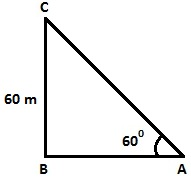Question

# A kite is flying at a height of $60m$ above the ground. The string attached to the kite is temporarily tied to a point on the ground. The inclination of the string with the ground is $60°$. Find the length of the string, assuming that there is no slack in the string.

Open in App
Solution

## Step 1: Draw the figure from the given data.From the given data, the figure isHere,$BC=$Height of the kite from the ground, $BC=60m$$AC=$Inclined length of the string from the ground and A is the point where string of the kite is tied.Step 2: Find the Length of the string from the ground i.e. the value of $AC$$\mathrm{sin}60°=BC/AC\phantom{\rule{0ex}{0ex}}⇒\frac{\surd 3}{2}=\frac{60}{AC}\phantom{\rule{0ex}{0ex}}⇒AC=40\surd 3m$Thus, the length of the string from the ground is $40\surd 3m.$Suggest Corrections1Similar questions